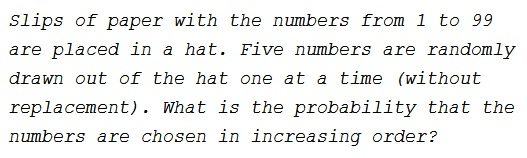# Probability of Increasing Sequence

### Problem### Answer

1/120. You can check the solution.

### Solution

It does not at all matter whether there are 99 slips of paper or just 5. The best way to approach the problem is to consider drawing just two numbers. So you've drawn two distinct numbers: what is the probability that the first one will be less than the second? Well, there are just two ways to draw two chosen numbers: the smallest either come first or last, meaning that the probability of drawing two numbers in their natural order is 1/2.

When it comes to five numbers. There are 120 permutations of five objects and thus 120 ways to draw a given set of five numbers. Only in one permutation out of 120 all five numbers follow in their natural order of magnitude.

### A Variant

Same setup, but this time we don't see the numbers drawn. We are told that they happen to be in increasing order. What is the probability that the first number drawn was a 1?

### Solution 1 to the Variant

\displaystyle\begin{align}&\frac{\text{# of ways to choose four other numbers when 1 has already been chosen}}{\text{# of ways to choose 5 numbers}}\\ &=\frac{{98\choose 4}}{{99\choose 5}}=\frac{5}{99}. \end{align}

### Solution 2 to the Variant

Let $A$ be the event that a $1$ is drawn. Let $B$ be the event that the numbers came out in increasing order. I asked for $P(A|B).$ But $A$ and $B$ are independent. So, $P(A|B)=P(A).$ Five numbers are drawn; the probability that one of them is $1$ is $\displaystyle \frac{5}{99}.$

### Acknowledgment

This is a problem from MIT Opencourseware, PROBLEMS ON "HIDDEN" INDEPENDENCE AND UNIFORMITY, 18.S34 (FALL 2007)

Michael Weiner came up with a variant of the problem. Solution 1 to the Variant problem is by Josh Jordan; Solution 2 is by Michael Weiner.Copyright © 1996-2018 Alexander Bogomolny

 64859548